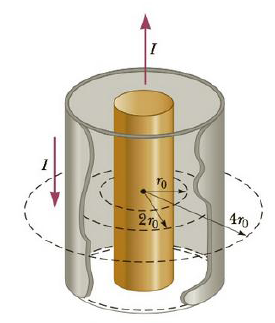Chapter 19, Problem 7CQ

Chapter
Section
Textbook Problem

Figure CQ19.7 shows a coaxial cable carrying current I in its inner conductor and a return current of the same magnitude in the opposite direction in the outer conductor. The magnetic field strength at r = r0 is Find the ratio B/B0, at (a) r = 2r0 and (b) r = 4r0.Figure CQ19.7

a)

To determine
The ratio BB0 at r=2r0 .

Explanation

Given info: The current in the inner conductor is I . The current in the outer conductor is in the opposite direction with same magnitude. For r=r0 the magnetic field is B0 .

Explanation:

The Amperes circuital law can be written as,

BΔl=μ0I

Segment of a closed path

B is the component of B parallel to the segment of length Δl

I is the current enclosed in the closed path

μ0 is the permeability of free space

For the circular path with r=r0 , the current enclosed inside the path is I . Hence using the Ampere law,

B0(2πr0)=μ0IB0=μ0I2πr0       (1)

For the circular path with r=2r0 , the current enclosed inside the path is I

b)

To determine
The ratio BB0 at r=4r0 .

Still sussing out bartleby?

Check out a sample textbook solution.

See a sample solution

The Solution to Your Study Problems

Bartleby provides explanations to thousands of textbook problems written by our experts, many with advanced degrees!

Get Started

What are the features of a healthy high-fat diet?

Understanding Nutrition (MindTap Course List)

Chylomicrons, a class of lipoprotein, are produced in the live. T F

Nutrition: Concepts and Controversies - Standalone book (MindTap Course List)

What three classes of lipids are found in membranes?

Chemistry for Today: General, Organic, and Biochemistry